# Optics

## Properties of light

### Wave particle duality:

Light is a wave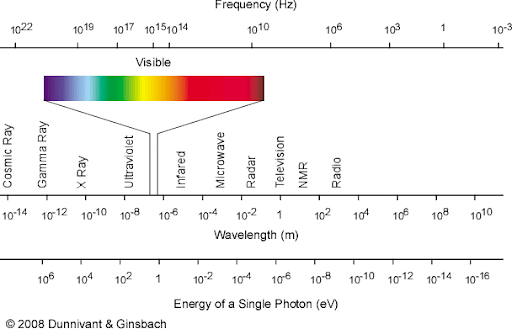Beer's law

Refraction

## Resolution

### Resolution: The ability to separate two objects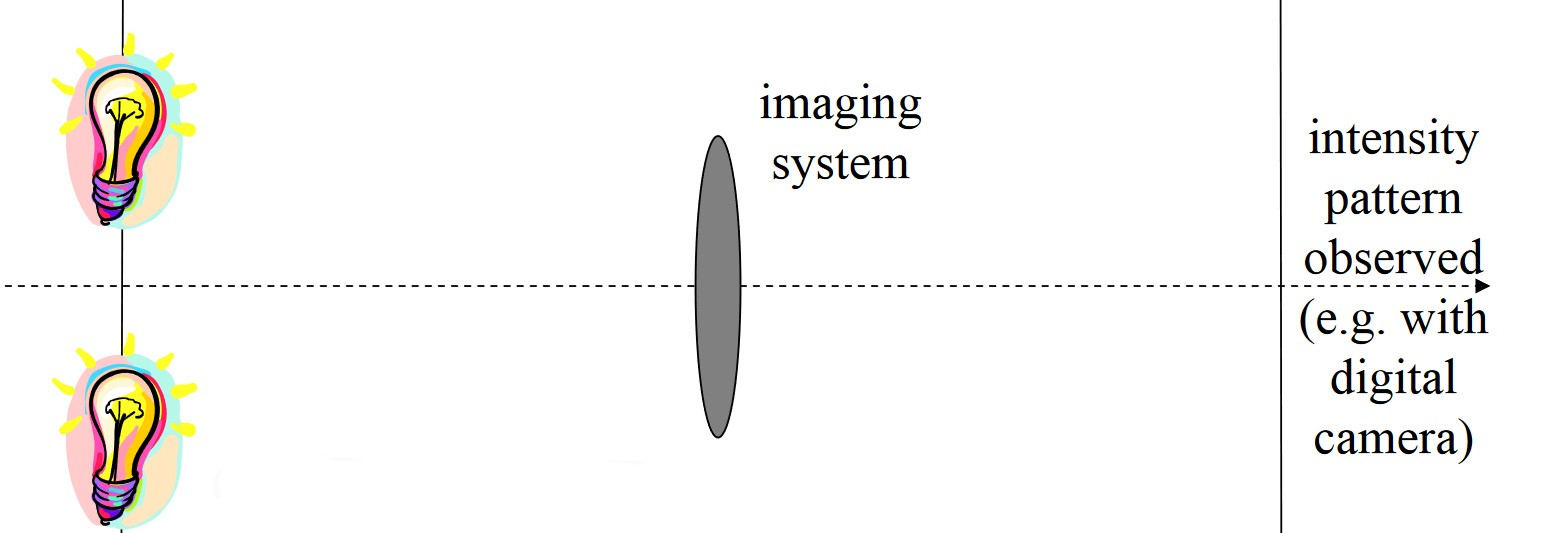### A definition of Numerical Aperture#### Fourier transform:

Transform from real space to frequency space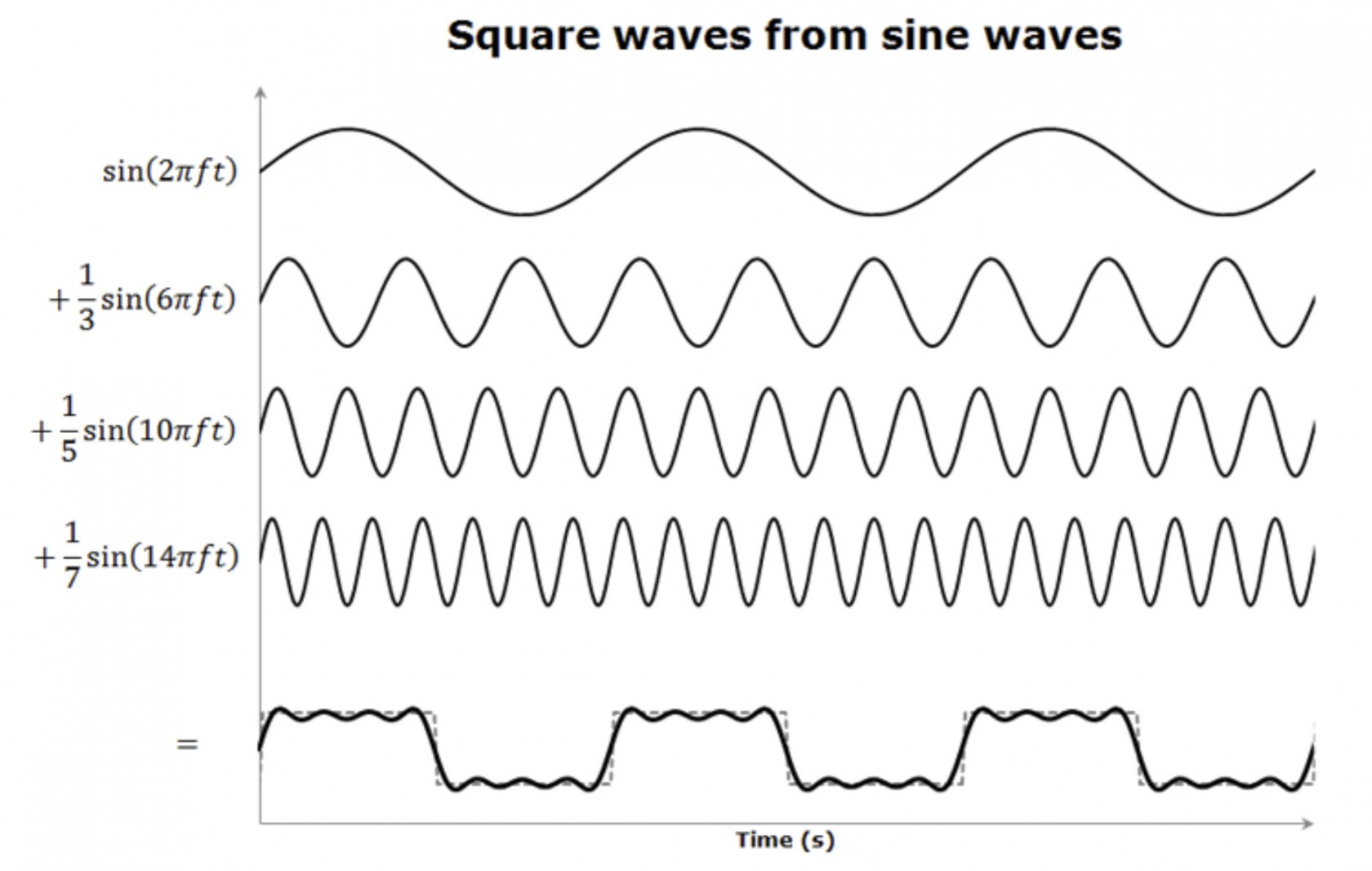Now we look at a real square wave and frequency space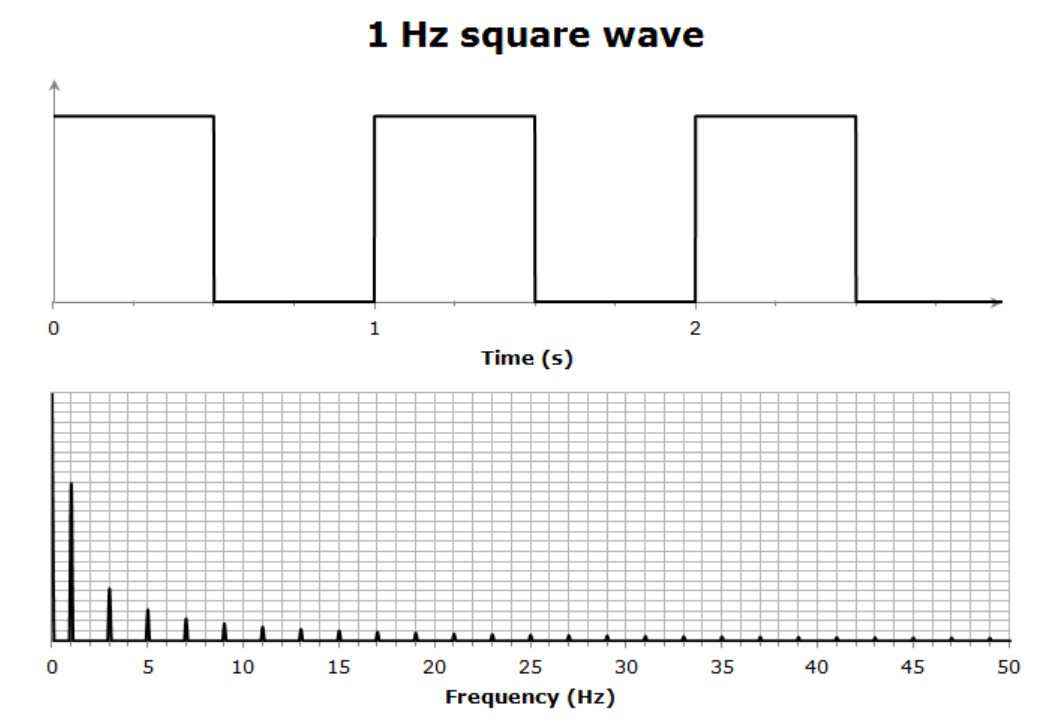### Look at a simple optical system:#### Mathematical prediction of the Point Spread Function (PSF)

on the left we have the mathematical point source know as a delta function.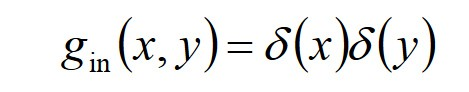were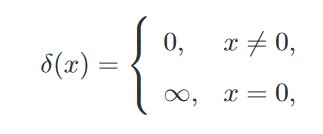the intensity at the Fourier plain can be found by taking the Fourier transform of this function.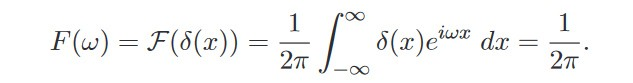or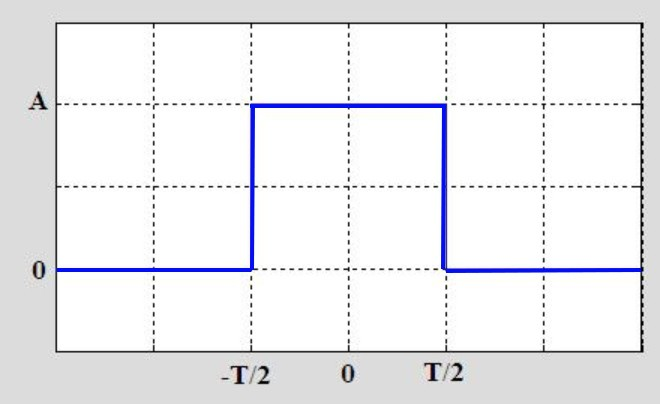this has the same intensity at all points inside the aperture and zero outside. The second lens is now taking a Fourier transform on a box function the width of the aperture.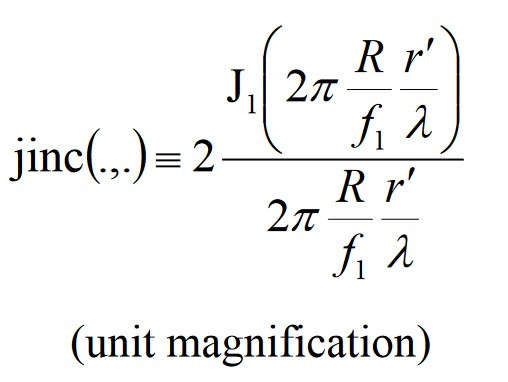or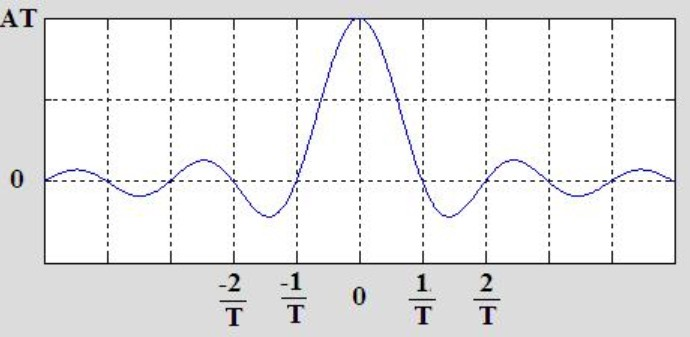Substituting in the definition for NA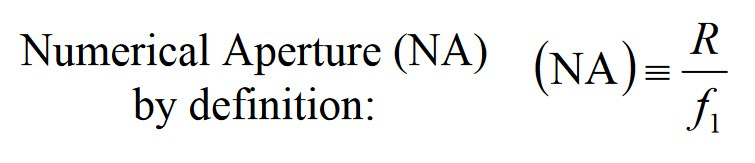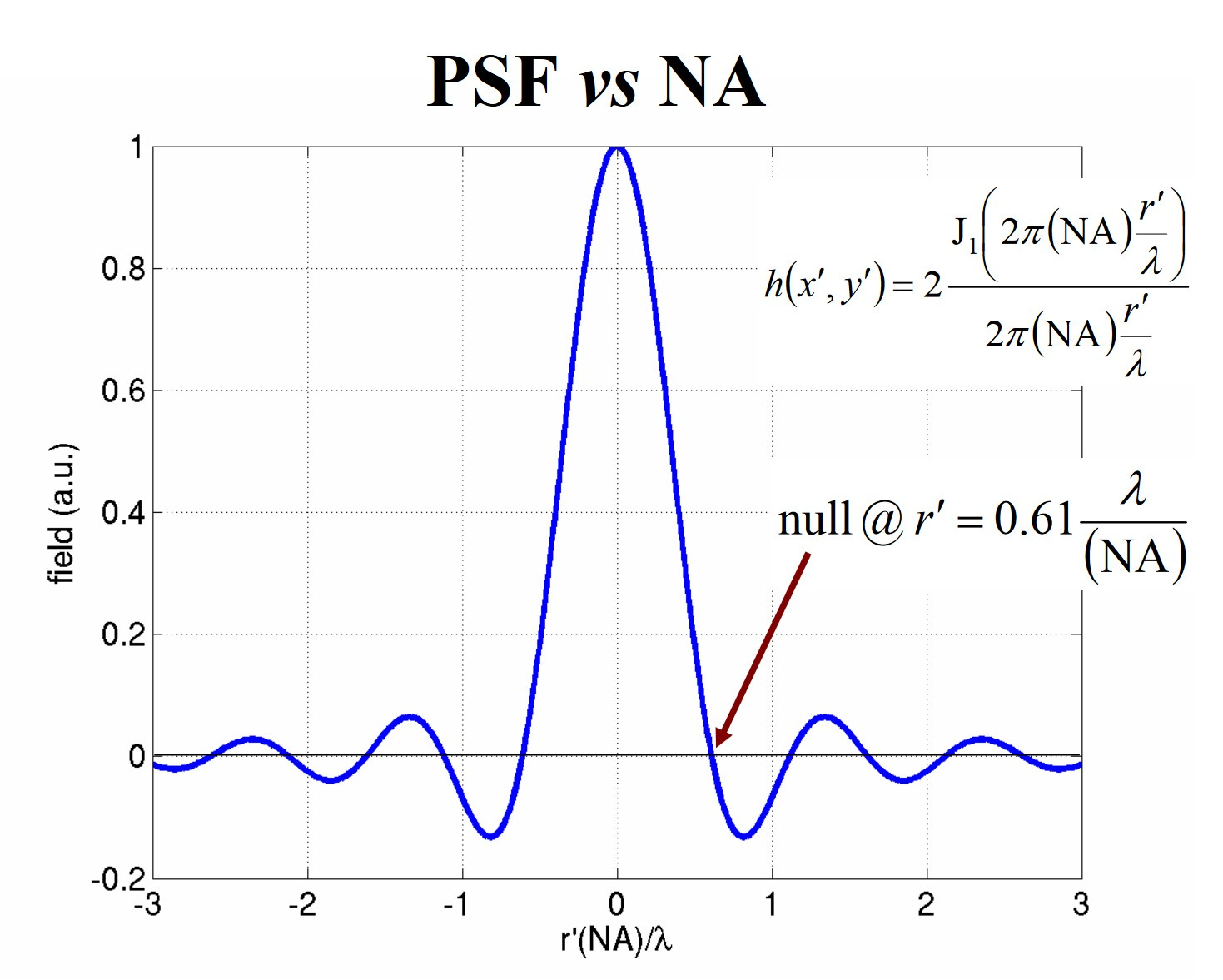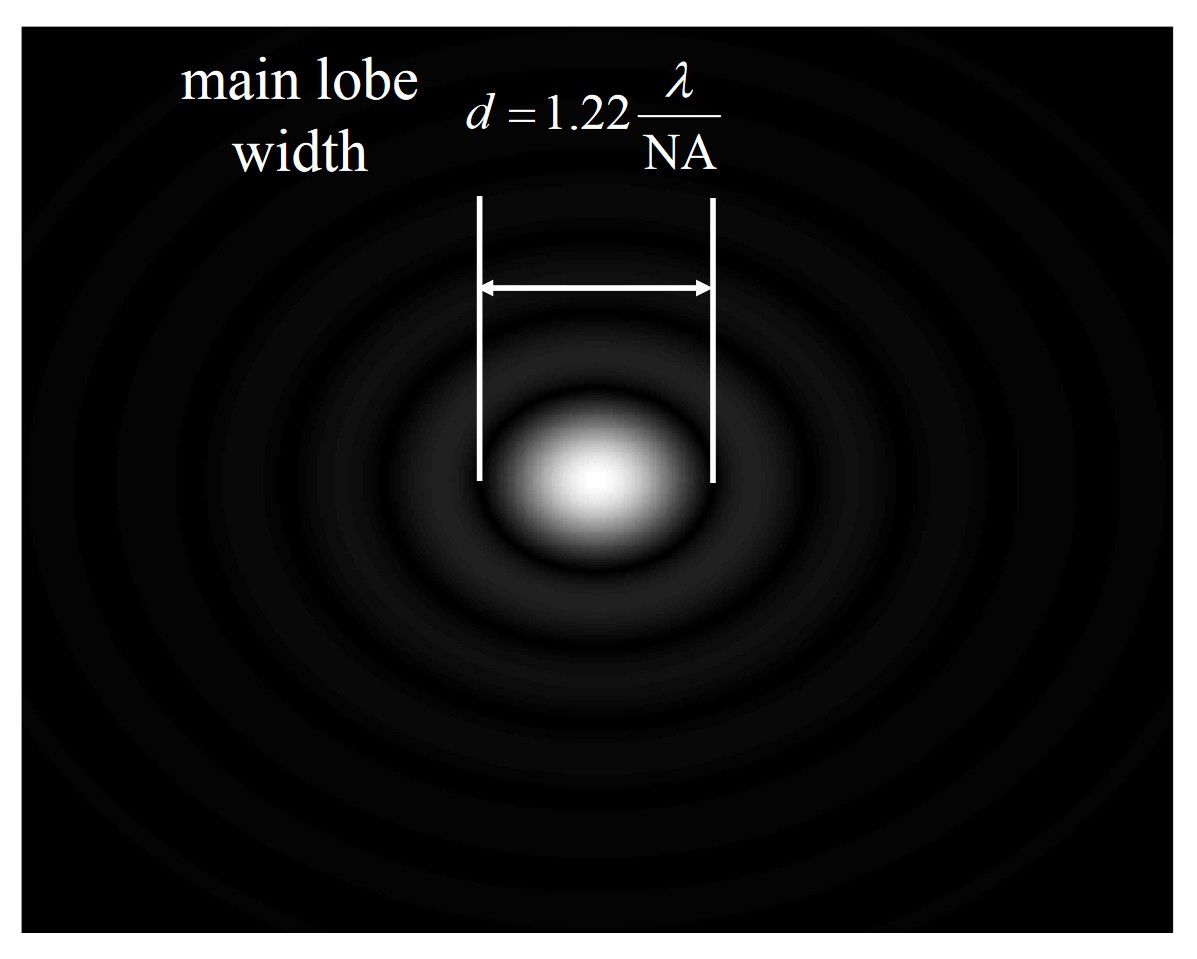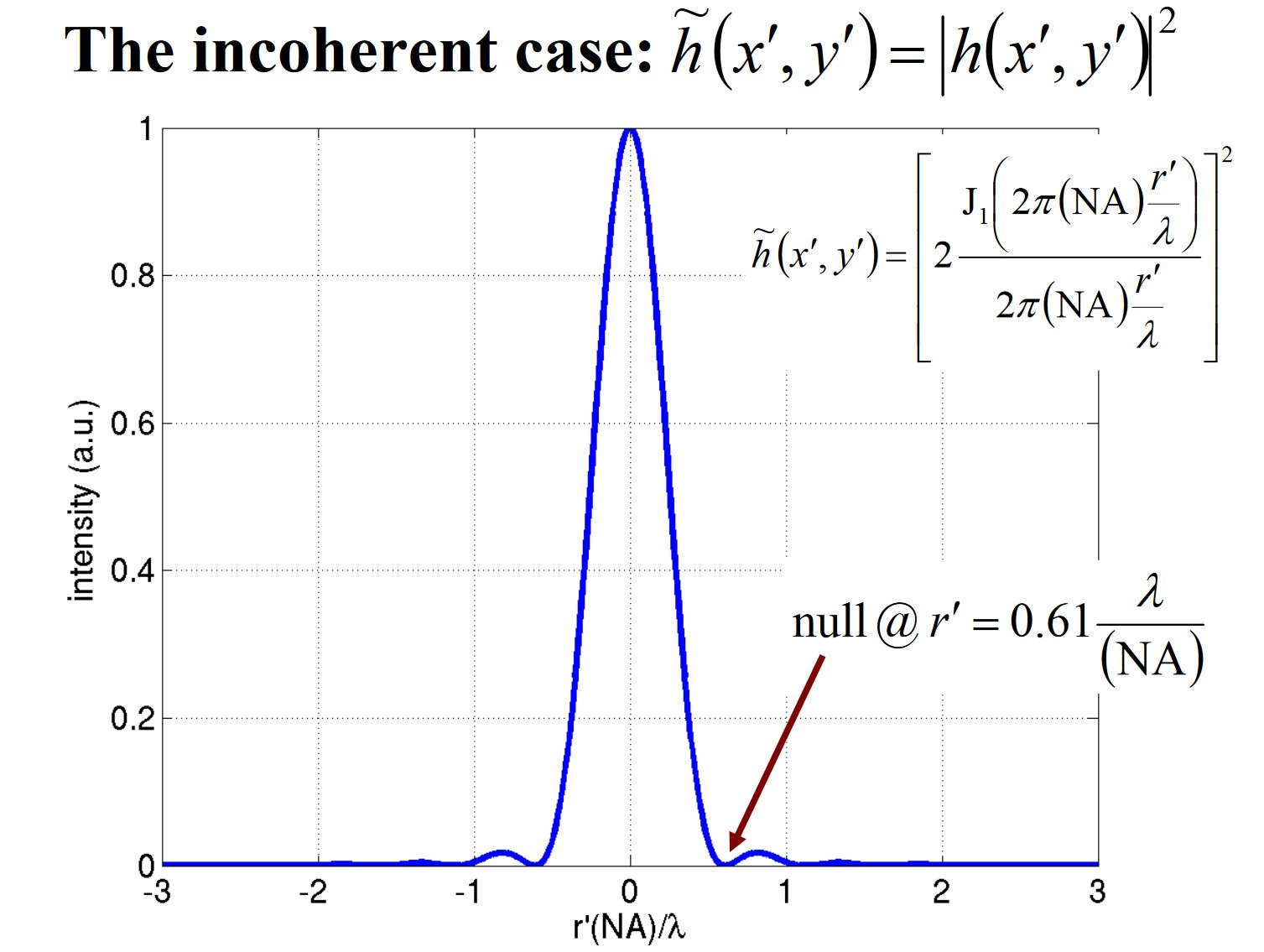### Now we go back to Resolution. How close together we can position two points and still distinguish them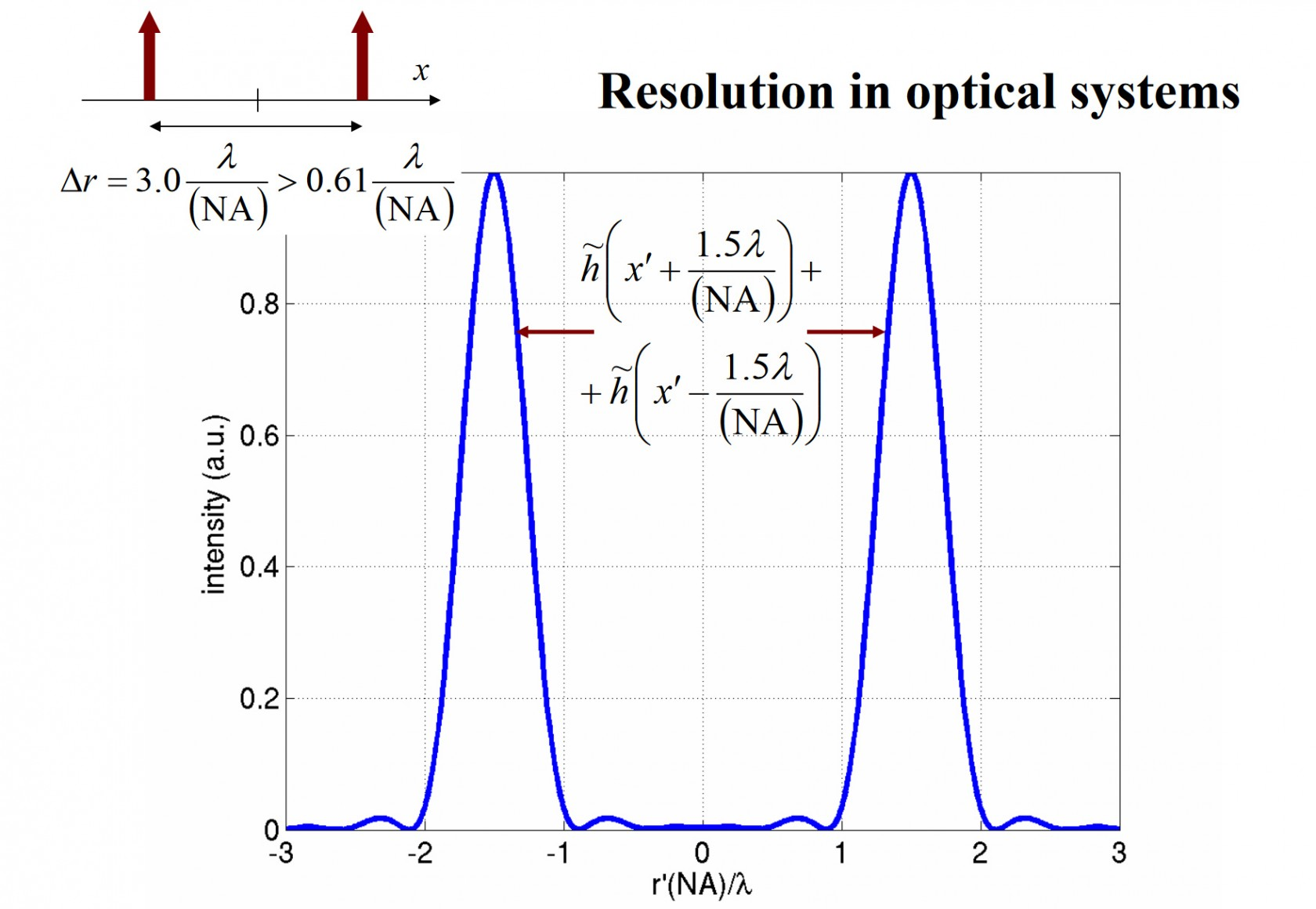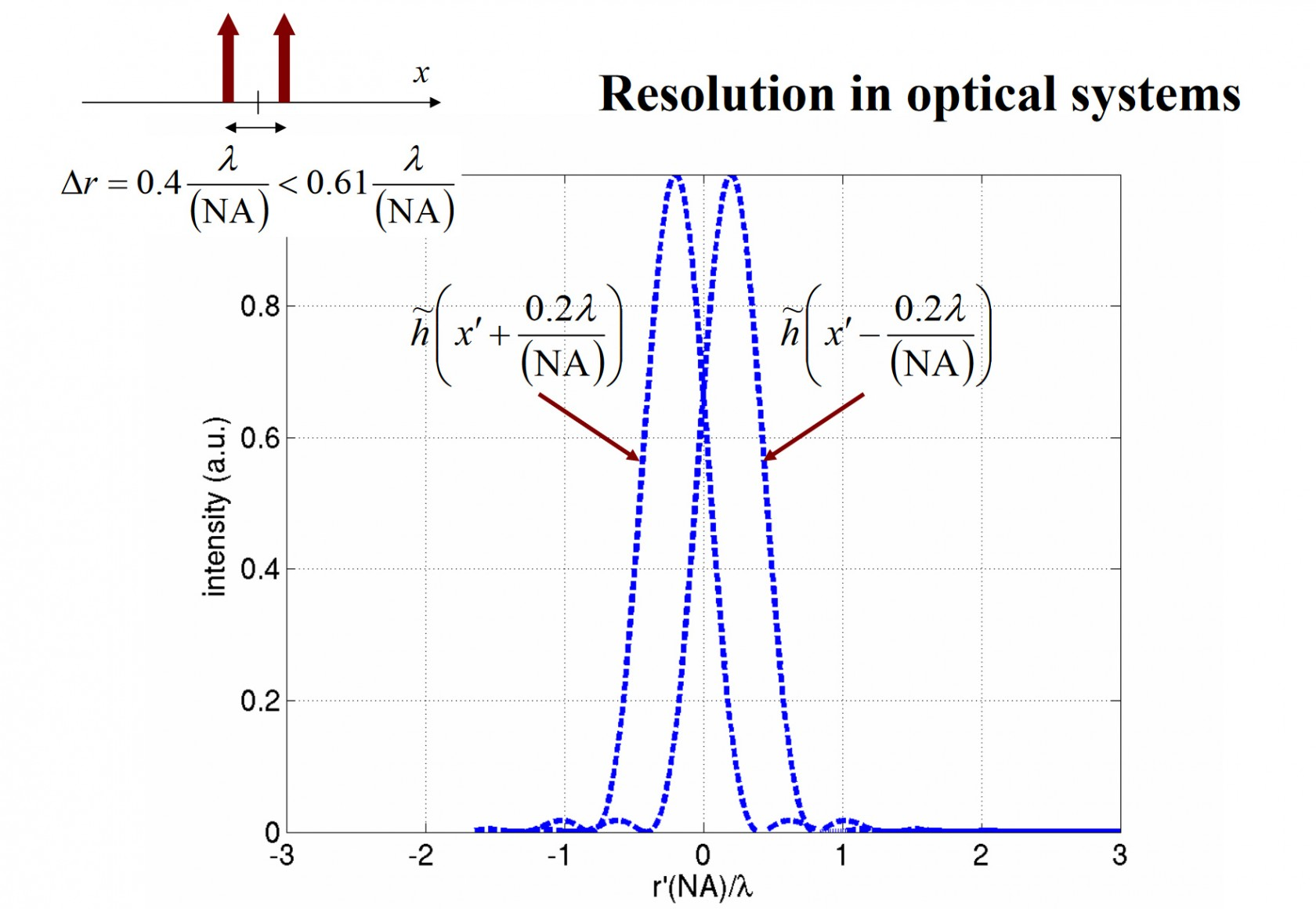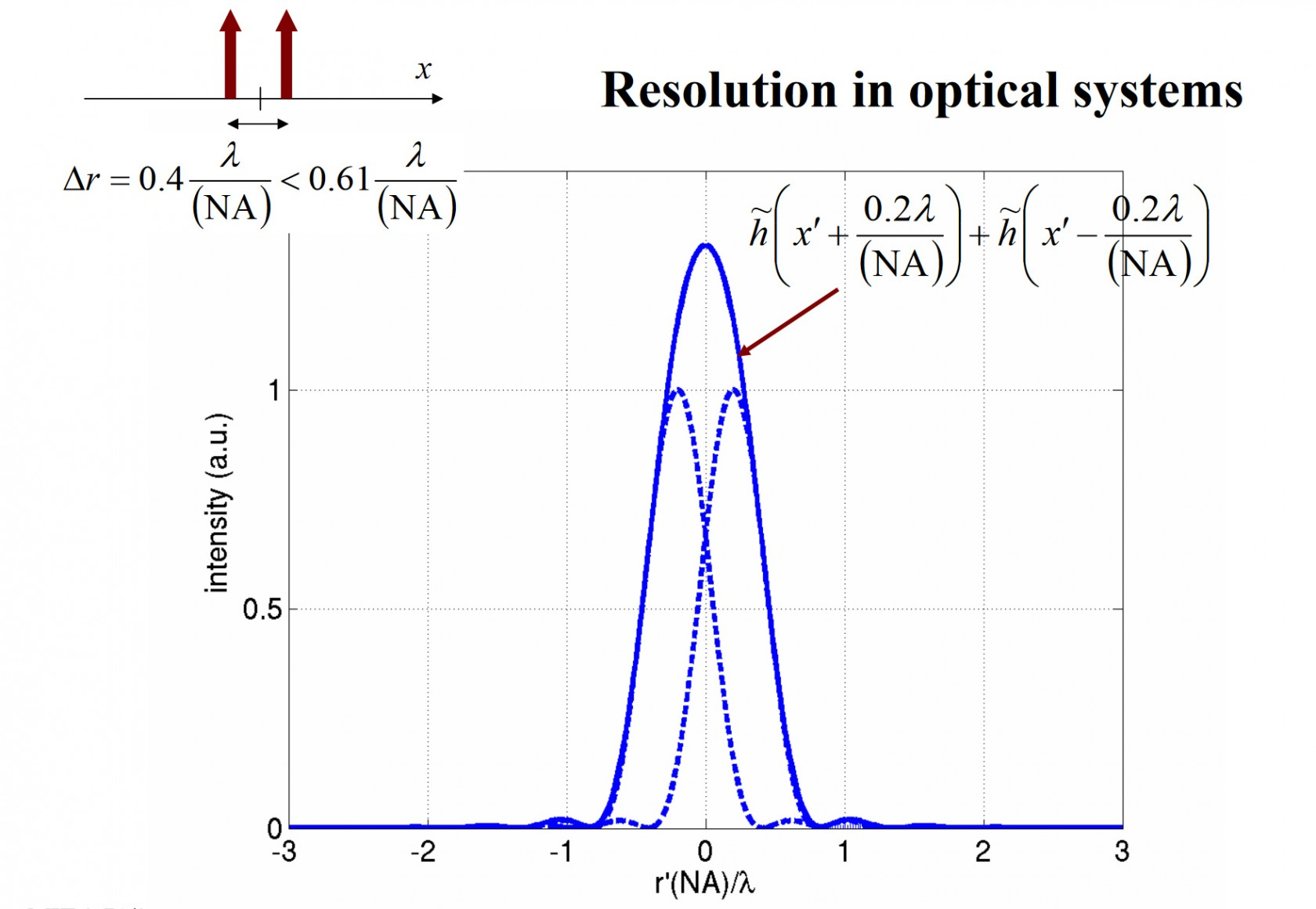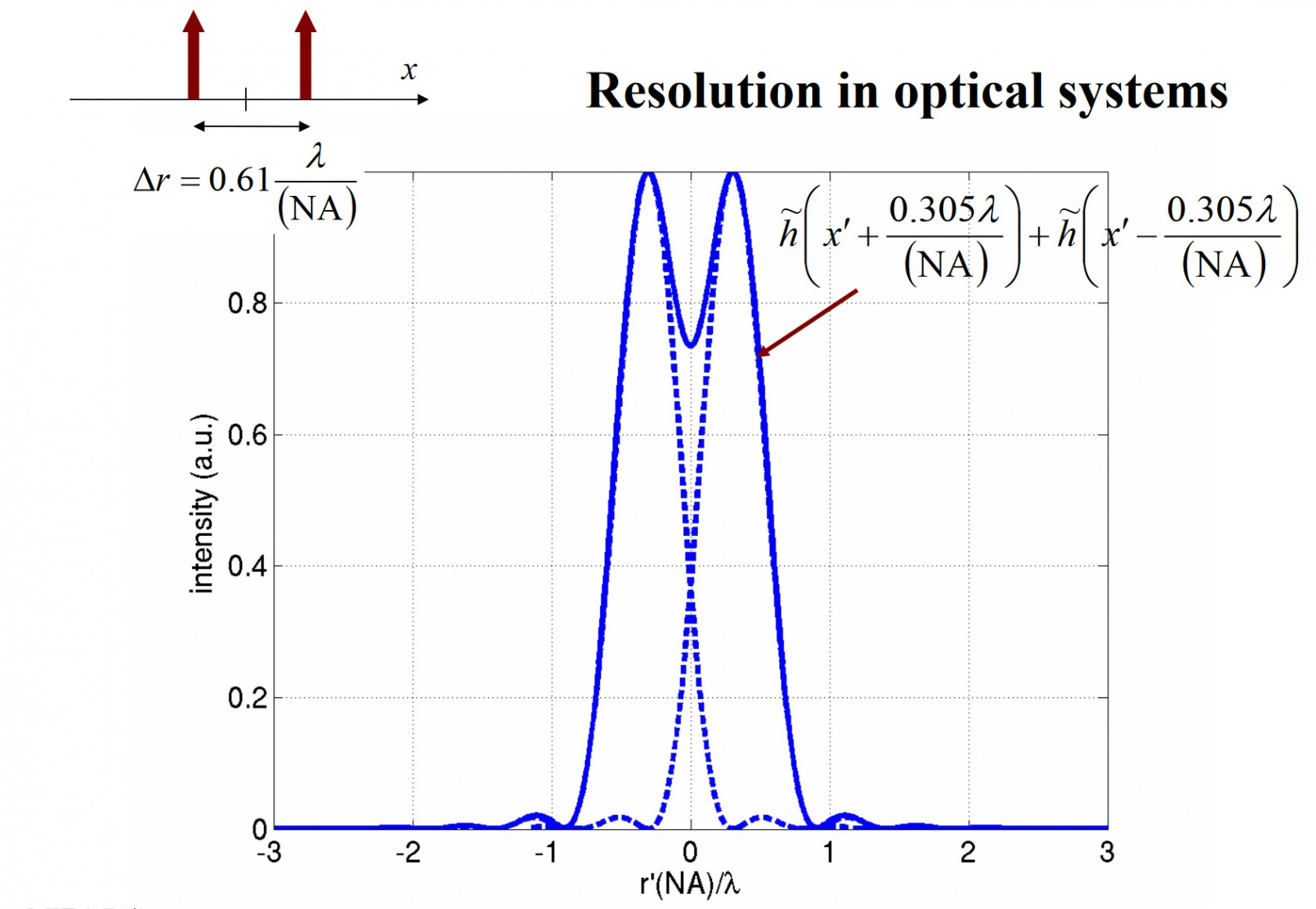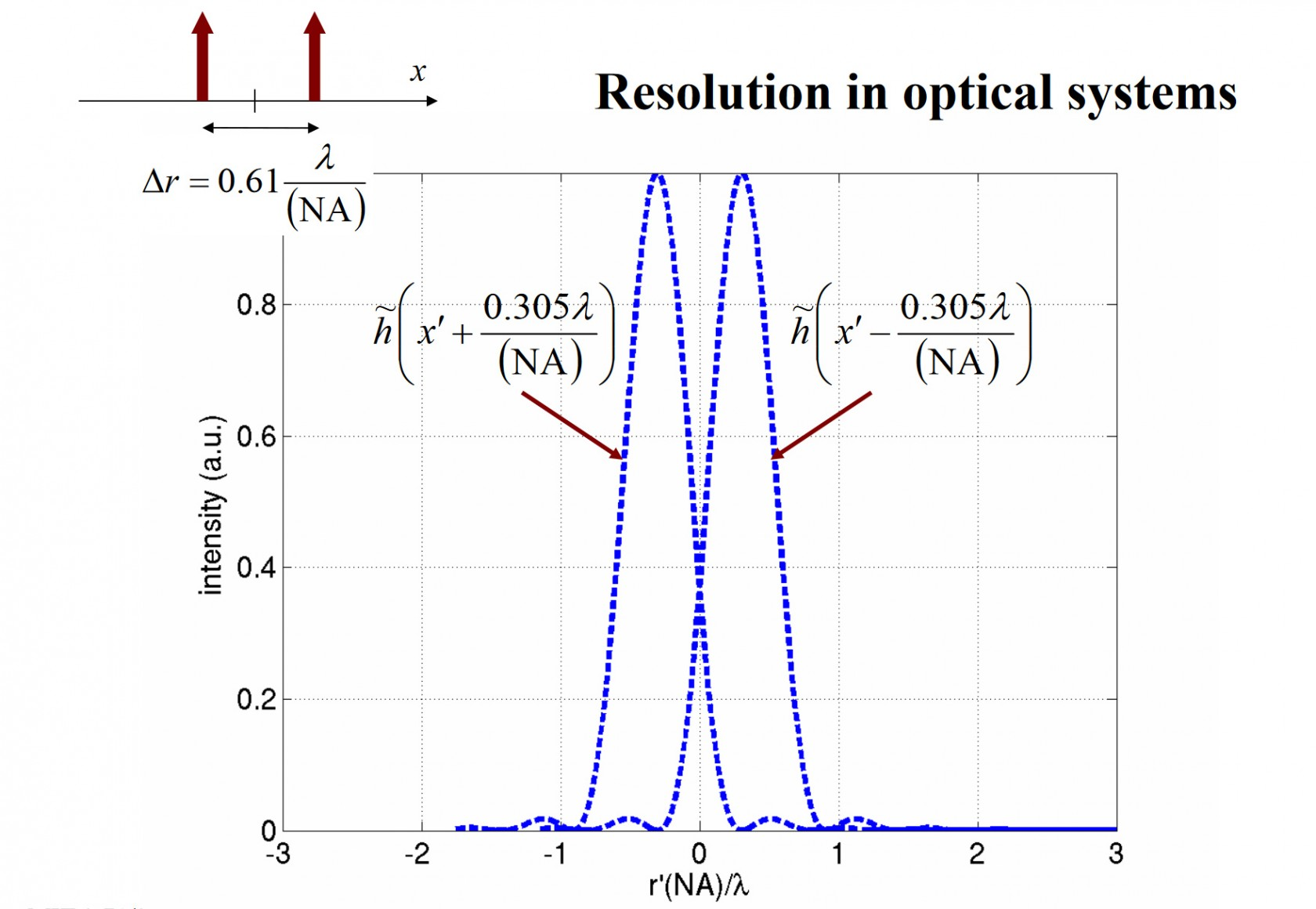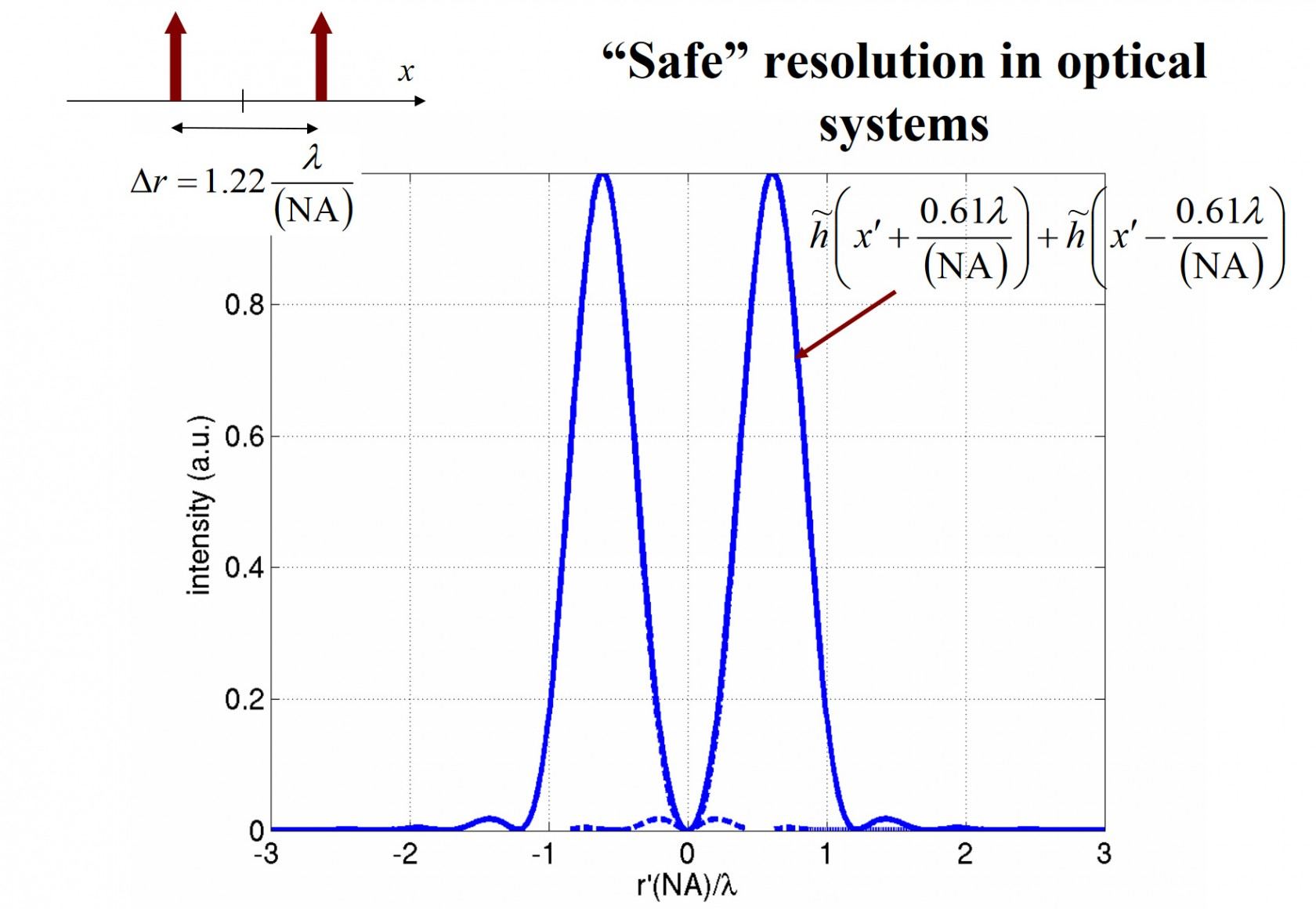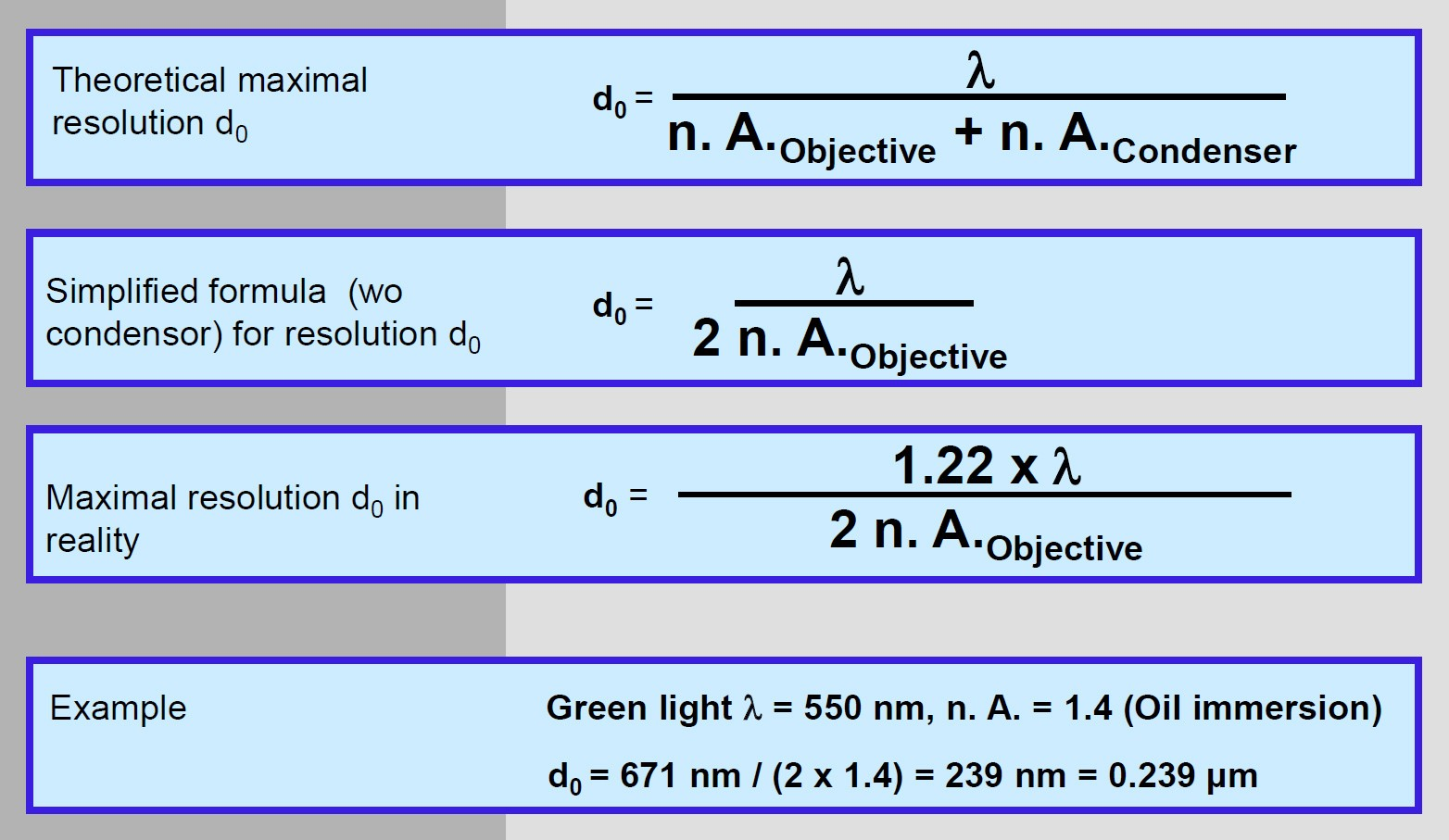More intuitive approach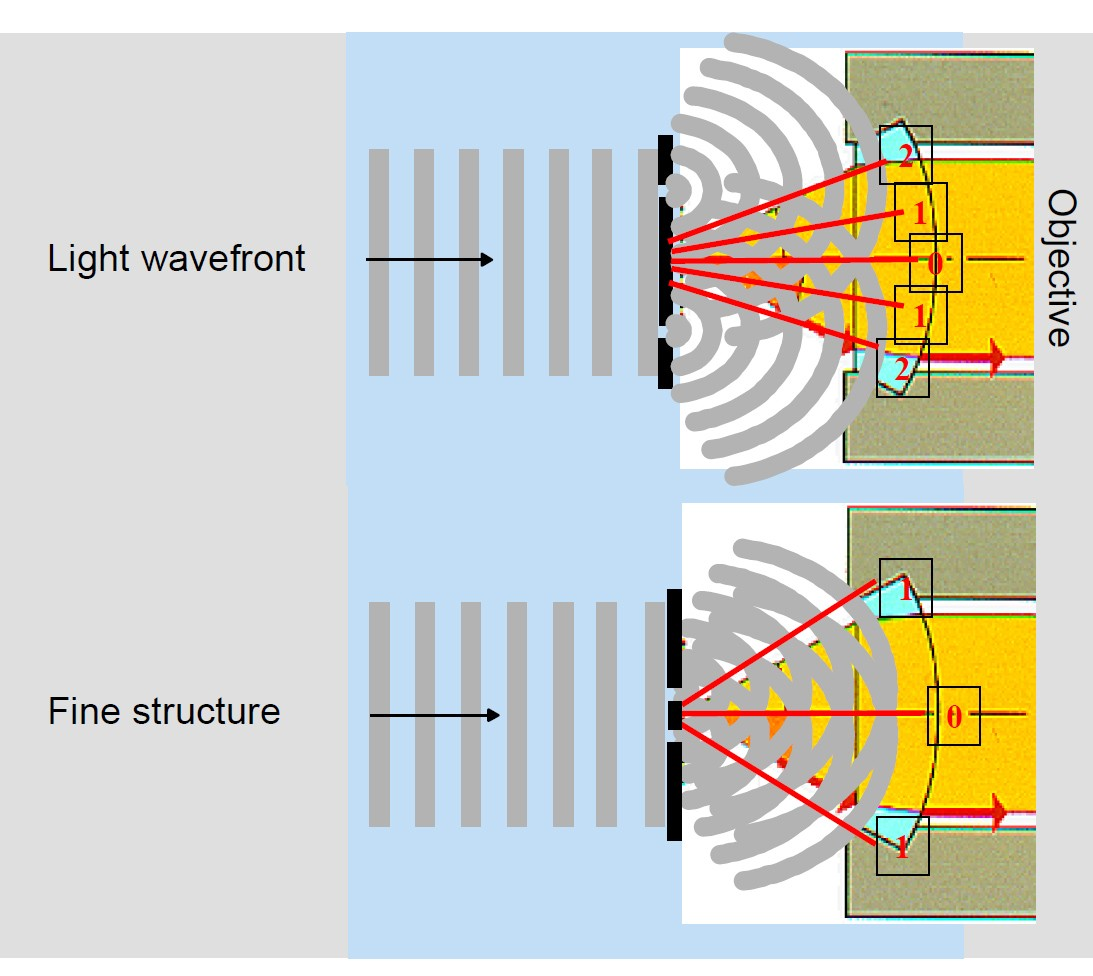Notes from:

http://web.mit.edu/2.710/Fall06/2.710-wk12-b-sl.pdf